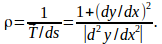## Radius of Curvature For a Curve y(x)

The position vector of a point on the curve is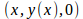so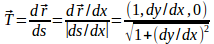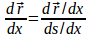where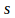is the length along the curve.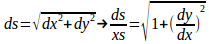and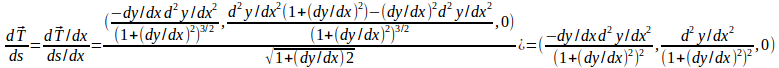The Frenet formula gives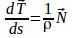where %rho is radius of the curvarure and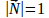hence